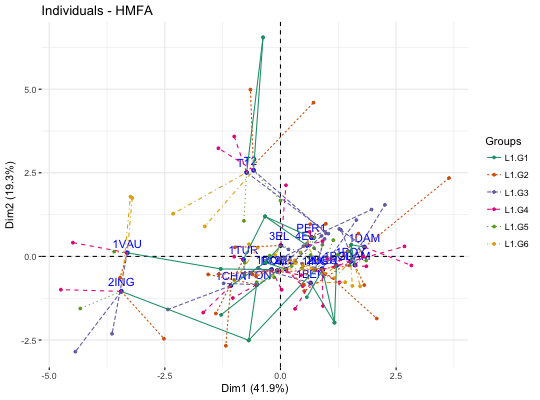Hierarchical Multiple Factor Analysis (HMFA) is, an extension of MFA, used in a situation where the data are organized into a hierarchical structure. fviz_hmfa() provides ggplot2-based elegant visualization of HMFA outputs from the R function: HMFA [FactoMineR].

• fviz_hmfa_ind(): Graph of individuals
• fviz_hmfa_var(): Graph of variables
• fviz_hmfa_quali_biplot(): Biplot of individuals and qualitative variables
• fviz_hmfa(): An alias of fviz_hmfa_ind()

fviz_hmfa_ind(X, axes = c(1, 2), geom = c("point", "text"), repel = FALSE,
habillage = "none", addEllipses = FALSE, shape.ind = 19,
col.ind = "blue", col.ind.sup = "darkblue", alpha.ind = 1,
select.ind = list(name = NULL, cos2 = NULL, contrib = NULL),
partial = NULL, col.partial = "group", group.names = NULL,
node.level = 1, ...)

fviz_hmfa_var(X, choice = c("quanti.var", "quali.var", "group"), axes = c(1,
2), geom = c("point", "text"), repel = FALSE, col.var = "red",
alpha.var = 1, shape.var = 17, col.var.sup = "darkgreen",
select.var = list(name = NULL, cos2 = NULL, contrib = NULL), ...)

fviz_hmfa_quali_biplot(X, axes = c(1, 2), geom = c("point", "text"),
repel = FALSE, habillage = "none",
title = "Biplot of individuals and qualitative variables - HMFA", ...)

fviz_hmfa(X, ...)

## Arguments

X
an object of class HMFA [FactoMineR].
axes
a numeric vector of length 2 specifying the dimensions to be plotted.
geom
a text specifying the geometry to be used for the graph. Allowed values are the combination of c("point", "arrow", "text"). Use "point" (to show only points); "text" to show only labels; c("point", "text") or c("arrow", "text") to show arrows and texts. Using c("arrow", "text") is sensible only for the graph of variables.
repel
a boolean, whether to use ggrepel to avoid overplotting text labels or not.
habillage
an optional factor variable for coloring the observations by groups. Default value is "none". If X is an HMFA object from FactoMineR package, habillage can also specify the index of the factor variable in the data.
logical value. If TRUE, draws ellipses around the individuals when habillage != "none".
shape.ind, shape.var
point shapes of individuals and variables, respectively.
col.ind, col.var
color for individuals, partial individuals and variables, respectively. Can be a continuous variable or a factor variable. Possible values include also : "cos2", "contrib", "coord", "x" or "y". In this case, the colors for individuals/variables are automatically controlled by their qualities ("cos2"), contributions ("contrib"), coordinates (x^2 + y^2 , "coord"), x values("x") or y values("y"). To use automatic coloring (by cos2, contrib, ....), make sure that habillage ="none".
col.ind.sup
color for supplementary individuals
alpha.ind, alpha.var
controls the transparency of individual, partial individual and variable, respectively. The value can variate from 0 (total transparency) to 1 (no transparency). Default value is 1. Possible values include also : "cos2", "contrib", "coord", "x" or "y". In this case, the transparency for individual/variable colors are automatically controlled by their qualities ("cos2"), contributions ("contrib"), coordinates (x^2 + y^2 , "coord"), x values("x") or y values("y"). To use this, make sure that habillage ="none".
select.ind, select.var
a selection of individuals and variables to be drawn. Allowed values are NULL or a list containing the arguments name, cos2 or contrib:
• name is a character vector containing individuals/variables to be drawn
• cos2 if cos2 is in [0, 1], ex: 0.6, then individuals/variables with a cos2 > 0.6 are drawn. if cos2 > 1, ex: 5, then the top 5 individuals/variables with the highest cos2 are drawn.
• contrib if contrib > 1, ex: 5, then the top 5 individuals/variables with the highest cos2 are drawn
partial
list of the individuals for which the partial points should be drawn. (by default, partial = NULL and no partial points are drawn). Use partial = "All" to visualize partial points for all individuals.
col.partial
color for partial individuals. By default, points are colored according to the groups.
group.names
a vector containing the name of the groups (by default, NULL and the group are named group.1, group.2 and so on).
node.level
a single number indicating the HMFA node level to plot.
...
Arguments to be passed to the function fviz() and ggpubr::ggpar()
choice
the graph to plot. Allowed values include one of c("quanti.var", "quali.var", "group") for plotting quantitative variables, qualitative variables and group of variables, respectively.
col.var.sup
color for supplementary variables.
title
the title of the graph

## Value

a ggplot

http://www.sthda.com/english/

## Examples

# Hierarchical Multiple Factor Analysis
# ++++++++++++++++++++++++
# Install and load FactoMineR to compute MFA
# install.packages("FactoMineR")
library("FactoMineR")
data(wine)
hierar <- list(c(2,5,3,10,9,2), c(4,2))
res.hmfa <- HMFA(wine, H = hierar, type=c("n",rep("s",5)), graph = FALSE)

# Graph of individuals
# ++++++++++++++++++++
# Color of individuals: col.ind = "#2E9FDF"
# Use repel = TRUE to avoid overplotting (slow if many points)
fviz_hmfa_ind(res.hmfa, repel = TRUE, col.ind = "#2E9FDF")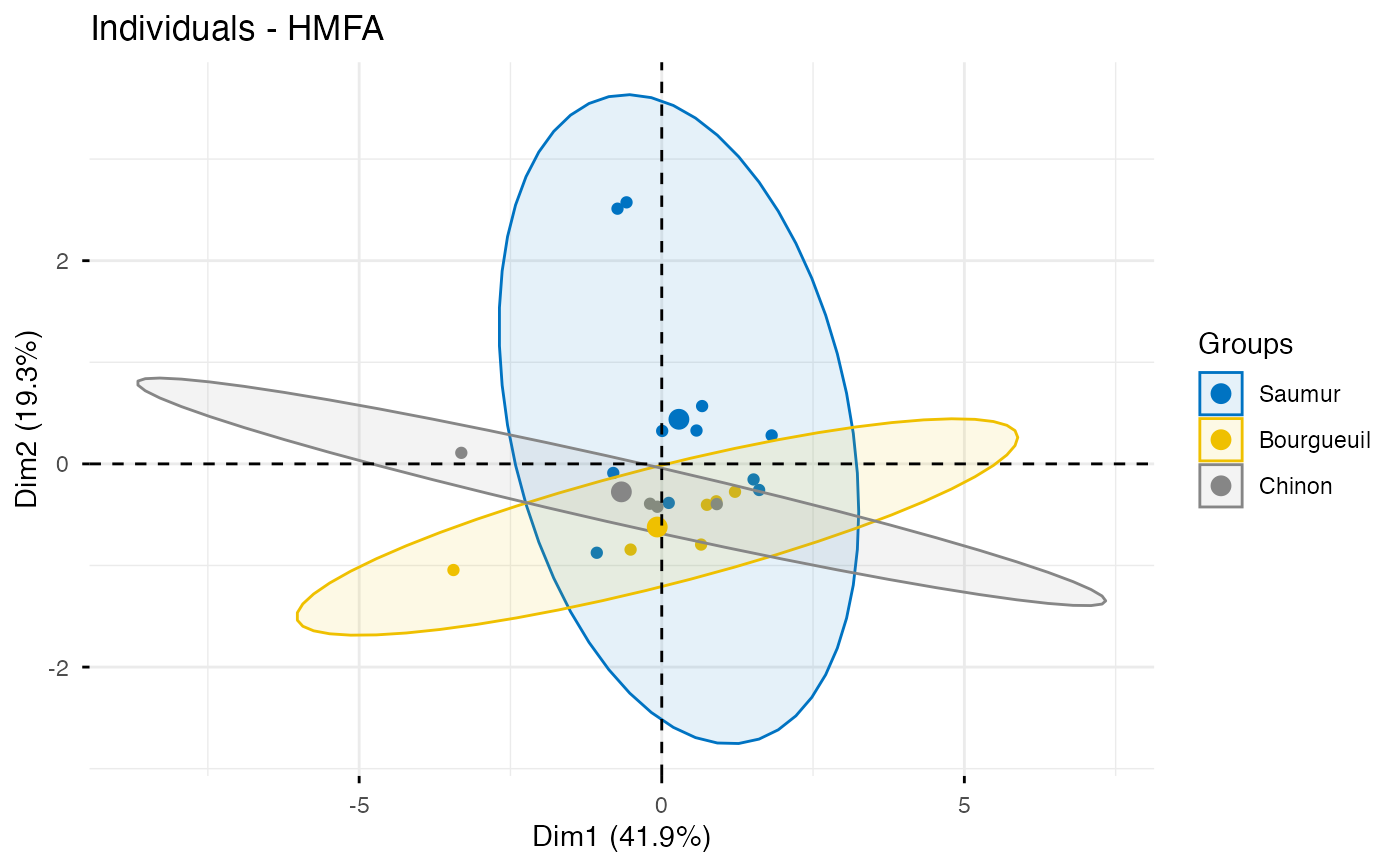# Color individuals by groups, add concentration ellipses
# Remove labels: label = "none".
# Change color palette to "jco". See ?ggpubr::ggpar
grp <- as.factor(wine[,1])
p <- fviz_hmfa_ind(res.hmfa, label="none", habillage=grp,
print(p)# Graph of variables
# ++++++++++++++++++++++++++++++++++++++++
# Quantitative variables
fviz_hmfa_var(res.hmfa, "quanti.var")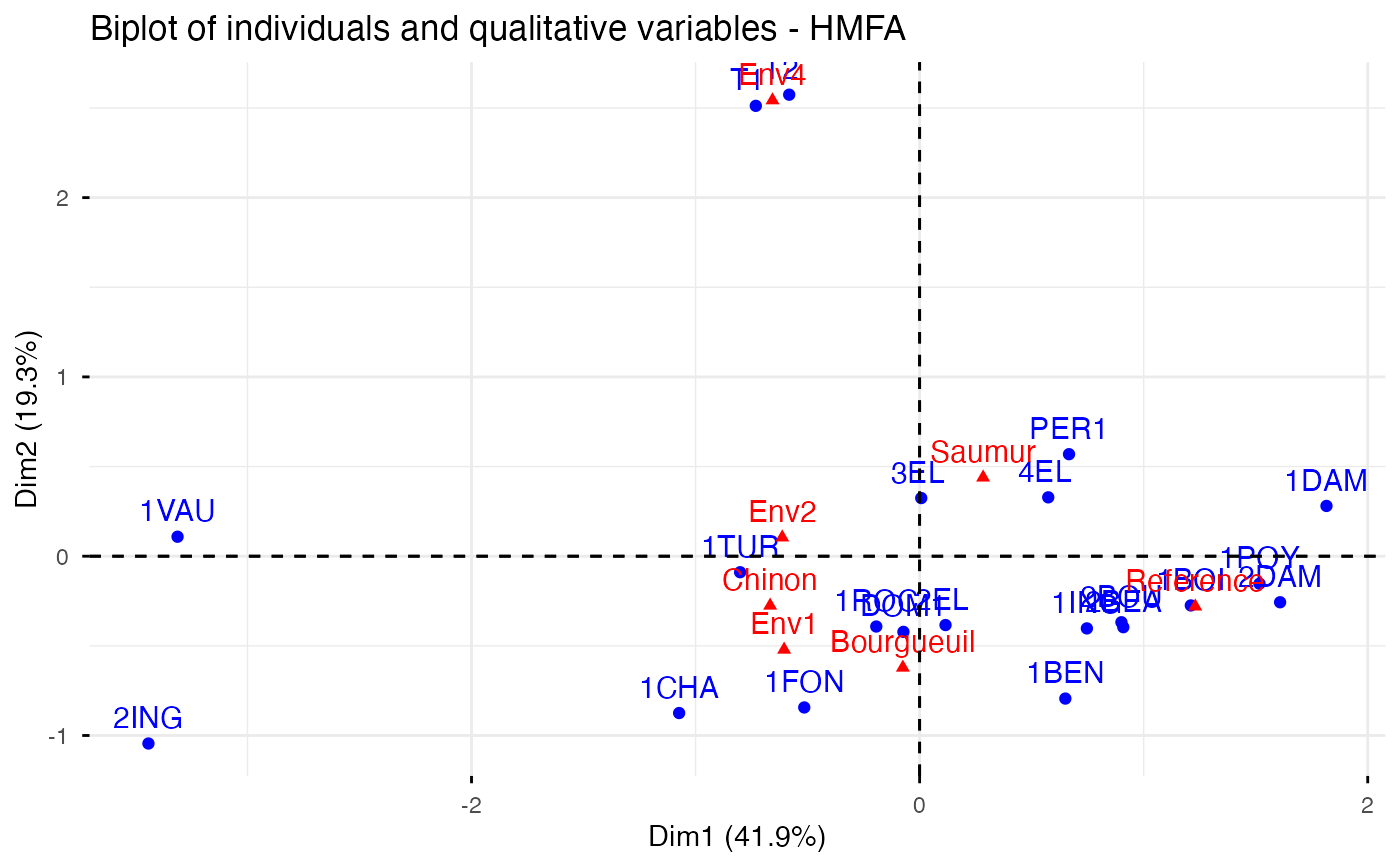# Graph of categorical variable categories
fviz_hmfa_var(res.hmfa, "quali.var")# Groups of variables (correlation square)
fviz_hmfa_var(res.hmfa, "group")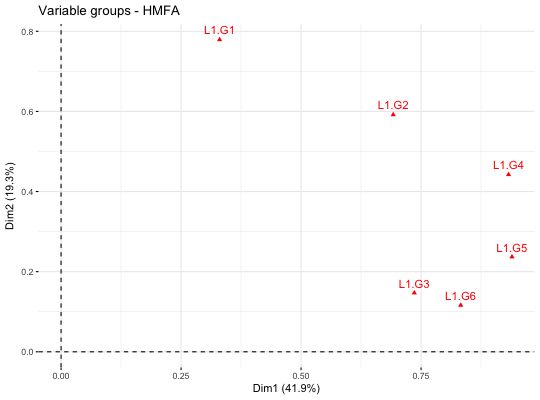# Biplot of categorical variable categories and individuals
# +++++++++++++++++++++++++++++++++++++++++++++++++++++++++
fviz_hmfa_quali_biplot(res.hmfa)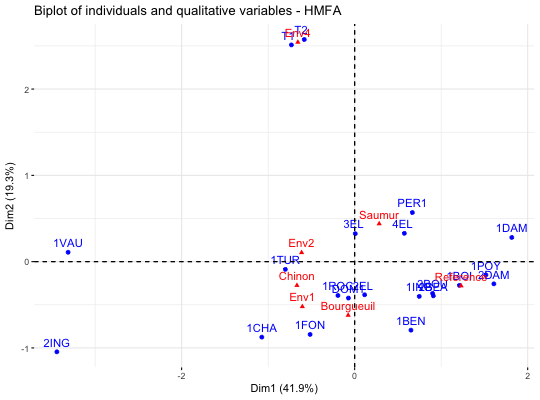# Graph of partial individuals (starplot)
# +++++++++++++++++++++++++++++++++++++++
fviz_hmfa_ind(res.hmfa, partial = "all", palette = "Dark2")